Question

Suppose that attendance at the concerts by the band “Keane” is a normally distributed random variable X with a mean of 18,500. You are told that P(X ≥ 15,000) = 0.6981. What are the two values of X that delineate the “82% middle pack” of this random variable?

A random variable has a population mean equal to 1,973 and population variance equal to 892,021. Your interest lies in estimating the population mean of this random variable. With that in mind, you take a representative sample of size 79 from the population of the random variable. You then use this sample data to calculate the sample average as an estimate for the population mean.

Required:
Using your knowledge about the central limit theorem (CLT), and assuming that the CLT has already “established itself” / “kicked in” when the sample size is 79, what is the probability that the sample average that you calculated will lie between 1,702 and 1,948?

1.linhdan

The two values of X that delineate the “82% middle pack” of this random variable are 9480 and 27520.

0.4017 = 40.17% probability that the sample average that you calculated will lie between 1,702 and 1,948.

Step-by-step explanation:

To solve the first question, we use the normal distribution, while for the second quetion, it is used with the central limit theorem.

Normal Probability Distribution:

Problems of normal distributions can be solved using the z-score formula.

In a set with mean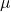and standard deviation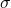, the z-score of a measure X is given by: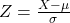The Z-score measures how many standard deviations the measure is from the mean. After finding the Z-score, we look at the z-score table and find the p-value associated with this z-score. This p-value is the probability that the value of the measure is smaller than X, that is, the percentile of X. Subtracting 1 by the p-value, we get the probability that the value of the measure is greater than X.

Central Limit Theorem

The Central Limit Theorem establishes that, for a normally distributed random variable X, with meanand standard deviation, the sampling distribution of the sample means with size n can be approximated to a normal distribution with meanand standard deviation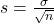.

For a skewed variable, the Central Limit Theorem can also be applied, as long as n is at least 30.

First question:

Mean of 18,500:

This means that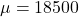You are told that P(X ≥ 15,000) = 0.6981.

This means that when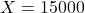, Z has a o-value of 1 – 0.6981 = 0.3019, which means that when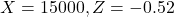. We use this to find. So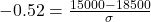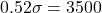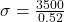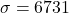What are the two values of X that delineate the “82% middle pack” of this random variable?

Between the 50 – (82/2) = 9th percentile and the 50 + (82/2) = 91st percentile.

9th percentile:

X when Z has a p-value of 0.09, so X when Z = -1.34.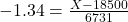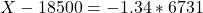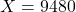91st percentile:

X when Z has a p-value of 0.91, so X when Z = 1.34.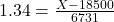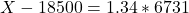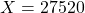The two values of X that delineate the “82% middle pack” of this random variable are 9480 and 27520.

Question 2:

A random variable has a population mean equal to 1,973 and population variance equal to 892,021.

This means that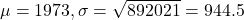Sample of 79:

This means that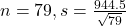What is the probability that the sample average that you calculated will lie between 1,702 and 1,948?

This is the p-value of Z when X = 1948 subtracted by the p-value of Z when X = 1702. So

X = 1948By the Central Limit Theorem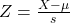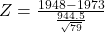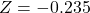has a p-value of 0.4071

X = 1702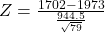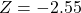has a p-value of 0.0054

0.4071 – 0.0054 = 0.4017

0.4017 = 40.17% probability that the sample average that you calculated will lie between 1,702 and 1,948.